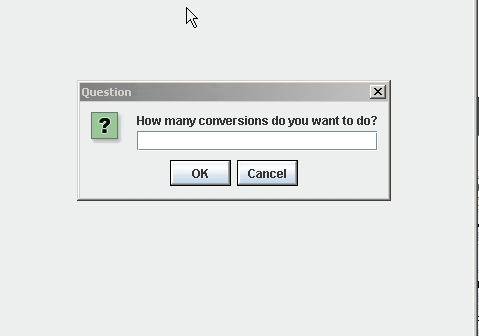ALice Loop Assignments1)  Centigrade to Fahrenheit Modification: Modify the Fahrenheit to centigrade project as follows.

1. Let the user do as many centigrade modifications as you want (like this link here)
2. Then use a loop to do that many conversions

2) Miles Per Gallon Modification: Modify the miles per gallon projec. In this one, ask the user how many times they want the MPG program to run and use a loop like this. .Due wed/Thurs the 17th/18th

1. Ask the user how many times, they want to do the MPG and use a loop like this
2. Then use a loop to do that many conversions

3) Sea Plane Loop-the-Loop : Create a world with a sea plane (from the Vehiclescollection).Create an infinite loop that causes the sea plane to do a circular loop the loop. Adjust the style and duration editing tags to make the animation fast, and as smooth as possible.

EOU)  Open assignment. Your open project must have each of the following criteraDue before Winter vacation

1. a variable
2. store the return value of a function into that variable
3. a loop of some kind